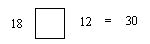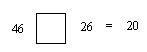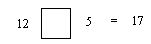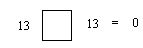Email us to get an instant 20% discount on highly effective K-12 Math & English kwizNET Programs!

#### Online Quiz (WorksheetABCD)

Questions Per Quiz = 2 4 6 8 10

### Grade 1 - Mathematics3.7 Missing Operand

 Method: If the result is a number is greater than the numbers on either side of the operand it is addition. If the result is a number less the number on the left side of the operand, it is subtraction. If the result is 0, the operand is subtraction. If the result is equal to the number on the left side of the operand, the operand is addition. Example: Find the missing operand: 12 ? 8 = 20 Here the result 20 is a number that is greater than the 12 and 8. Therefore the operand is addition. Answer: + Example: 12 ? 3 = 9 Here the result is a number less the number on the left side of the operand, it is subtraction. Answer: - Example: 12 ? 12 = 0 Here the result is 0, therefore the operand is subtraction. Answer: - Example: 12 ? 0 = 12 If the result is equal to the number on the left side of the operand, it is addition. Answer: + Directions: Answer the following questions. Also write at least ten examples of your own.
 Q 1: Find the missing operand.-+ Q 2: Find the missing operand.+- Q 3: Find the missing operand.+- Q 4: Find the missing operand.-+ Q 5: Find the missing operand.-+ Q 6: Find the missing operand.-+ Q 7: Find the missing operand.+- Q 8: Find the missing operand.-+ Question 9: This question is available to subscribers only! Question 10: This question is available to subscribers only!